MORE IN Circuits and Transmission Lines
MU Electronics and Telecom Engineering (Semester 3)
Circuits and Transmission Lines
December 2013
Total marks: --
Total time: --
INSTRUCTIONS
(1) Assume appropriate data and state your reasons
(2) Marks are given to the right of every question
(3) Draw neat diagrams wherever necessary

1 (a) Determine the y-parameters of the network shown in Fig. 1(a)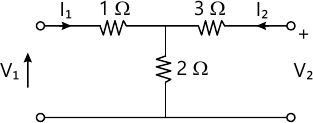5 M
1 (b) Test if F(s)=s6+2s5+3s4+4s3+3s2+2s+1 is a Hurwitz polynomial
5 M
1 (c) Both the coils connected in series have self inductance of 40 mH. The total inductance of the circuit is found to be 120 mH. Determine the (i) mutual inductance between the coils and (ii) the coefficient of coupling
5 M
1 (d) Find Foster I and II, and Cauer I and II circuit for the driving point damittance Y(s)=s+1
5 M

Solve any four questions :-
2 (a) Find the Thevenin equivalent across the terminal XY for the circuit shown in Fig. 2(a) using mesh matrix method.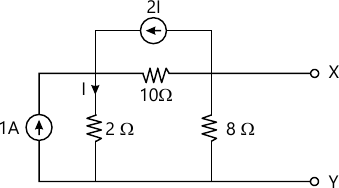10 M
2 (b) Find the magnitude of the controller source in the circuit shown in fig 2(b) by node analysis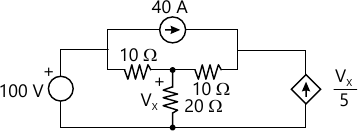5 M
2 (c) Check if the following polynomials are Hurwitz polynomials
(i) s2+8 (ii) (s+2)3
5 M

3 (a) "Synthesis the driving point function[ Fleft(s ight)=frac{left(s^2+1 ight)left(s^2+9 ight)}{sleft(s^2+4 ight)} ] when F(s) is a driving point
(i) impedance (ii) admittance. Test if the circuit obtained are canonic"
10 M
3 (b) Find the voltage transfer function of a loded two port network N in terms of the y-parameters (y11, y12, y21, y22) of the network N and load admittance YL
5 M
3 (c) The parameters of a transmission line are : G=2.25 m&Mho;/km, R=65Ω/km, L=1.6 mH/km, C=0.1 μF/km. Find Z0 and γ at a frequency of 1 GHz.
5 M

4 (a) Determine the ABCD parameters of the network shown in Fig 4(a)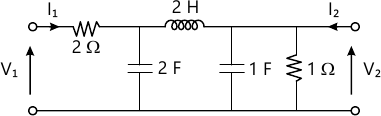10 M
4 (b) Determine the current transfer function for the circuit shown in Fig 4(b) first finding the Norton equivalent across XY and then Thevenin equivalent across AB under the conditions ω2L1C12L2C2=1.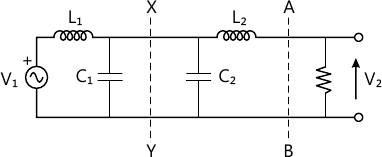5 M
4 (c) "Test if [ Fleft(s ight)=frac{s^3+6s^2+7s+3}{s^2+2s+1} ] is a Positive Real Function"
5 M

5 (a) The network shown in Fig 5(a) attains steady-state with the switch K open. At t=0, the switch is closed. Determine i1(0+), i2(0+), i3(0+).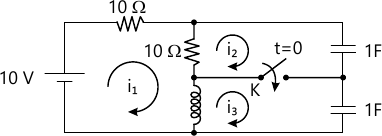10 M
5 (b) In the circuit shown in Fig 5(b) the two coupled coils have neglibible resistance. Find the current I2 when the input vi=10√2 sin 5000 t V. Is the circuit reciprocal?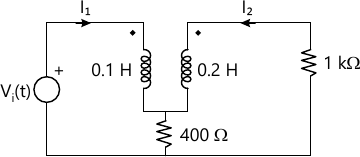5 M
5 (c) A generator of 1 V, 1 Khz supplies power to a 1000 km open write terminated in Z0 having the following parameters.
R=10.4Ω/km
L=3.97mH/km
G=0.8 μ&Mho;/km
C=0.00835 μF/km
Calculate the power dilivered at the receiving end.
5 M

6 (a) Why impedance matching required?
Draw the following normalized quantities on the Smith chart
(i) (1+j1)Ω
(ii) (2-j1)Ω
(iii) (0.5)Ω
(iv) (j0.5)Ω
10 M
6 (b) Find Z(s) for the circuit shown in Fig 6(b)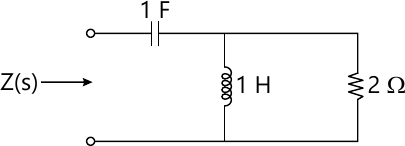5 M
6 (c) Find current i(t) through R in the circuit shown in Fig 6(c) using Laplace transform. Why is the frequency domain method preferred to the classical time domain method ?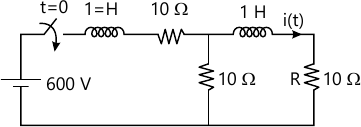5 M

More question papers from Circuits and Transmission Lines